# Evaporators (Part - 3) Chemical Engineering Notes | EduRev

## Chemical Engineering : Evaporators (Part - 3) Chemical Engineering Notes | EduRev

The document Evaporators (Part - 3) Chemical Engineering Notes | EduRev is a part of the Chemical Engineering Course Heat Transfer for Engg..
All you need of Chemical Engineering at this link: Chemical Engineering

9.7 Enthalpy Balance

9.7.1 Single effect evaporator
The latent heat of condensation of the steam is transferred to the boiling solution through the heating surface in order to vaporize the water. Thus, two enthalpy balances are required one for the liquid and another for the steam.

The following assumptions are required, in order to make the enthalpy balance,

1. Flow of non-condensable is negligible
2. The superheat and sub-cooling (see fig. 9.4) of the condensable steam is negligible
3. No solid precipitates out from the concentrating solution

The enthalpy balance for the steam side is,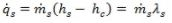Where,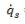= rate of heat transfer through heating surface from steam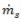=  flow rate of steam
λs = latent heat of condensation of steam
hs = specific enthalpy of steam
hc = specific enthalpy of condensate

Enthalpy balance for the liquid side is (eq.9.3),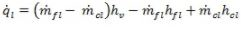(9.3)

Where,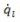=  rate of heat transfer from heating surface to the liquid
hv = specific enthalpy of vapour
hcl = specific enthalpy of concentrated liquid
hfl = specific enthalpy of liquid feed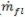=  flow rate of liquid feed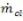= flow rate of concentrated liquid

The enthalpy balance at steam side and liquid side will be same in the absence of any heat loss (eq.9.4). Thus,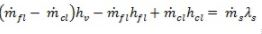(9.4)

The area of heat transfer A can be calculated from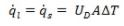When ΔT = (Tb - Tc);
Tb = Saturated temperature of steam in the shell
Ts = Boiling point of the solution at the prevailing pressure
UD = Overall coefficient (dirty)

9.7.2 Effect of heat of dilution

Most of the solutions when mixed or diluted at constant temperature do not give significant heat effect. It is generally true for organic solutions (like sugar). However, many of the inorganic solutions (like sulfuric acid, potassium hydroxide, calcium carbonate etc.) evolve significant heat on dilution. Therefore, an equivalent amount of heat is required (in addition to the latent heat of vaporization), when dilute solutions of these inorganic chemicals are concentrated. Enthalpy-concentration diagram are helpful in order to find the enthalpy of the solution at different concentration of these chemicals in the solution.

9.7.3 Multiple effect evaporators
The steam goes into I-effect and heat the solution by the latent heat of condensation. If the heat required to boil the feed is negligible, it follows that practically all this heat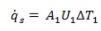must appear as latent heat in the vapor that leaves the I-effect and enter into II-effect as steam. The temperature of the condensate leaving the II-effect will be very near the temperature T1 of the vapors from the boiling liquid in the I-effect. Thus, in steady state operation all the heat that was expanded in creating vapor in the I-effect must be given by when this same vapor condenses in the II-effect and so on.

The heat delivered into the II-effect will be,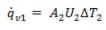The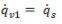Similarly, for III-effect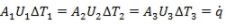(9.5)

It can be seen (eq. 9.5) that the temperature drops in a multiple effect evaporator is approximately inversely proportional to the heat-transfer coefficient.

The total available temperature drop will be given by eq.9.6,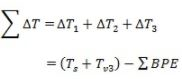(9.6)

where,
Ts : Steam temp. (I-effect); Tv3 : Vapor temperature leaving III-effect
BPE : boiling point elevation in the solution in various effects

Most of the solutions when mixed or diluted at constant temperature do not give significant heat effect. It is generally true for organic solutions (like sugar). However, many of the inorganic solutions (like sulfuric acid, potassium hydroxide, calcium carbonate etc.) evolve significant heat on dilution. Therefore, an equivalent amount of heat is required (in addition to the latent heat of vaporization), when dilute solutions of these inorganic chemicals are concentrated. Enthalpy-concentration diagram are helpful in order to find the enthalpy of the solution at different concentration of these chemicals in the solution.

Illustration
A triple effect forward feed evaporator is used to concentrate a liquid which has marginal elevation in boiling point. The temperature of the stream to the first effect is 105°C, and the boiling point of the solution within third effect is 45°C. The overall heat transfer coefficients are,

2,200 W/m2: in the Ι-effect,
1,800 W/m2: in the ΙΙ-effect,
1,500 W/m2: in the ΙΙΙ-effect.

Find out at what temperatures the fluid boils in the Ι and ΙΙ effects.
Solution:
Assumptions
1. We may assume that there is no elevation in boiling point in the evaporators.
2. Area of all the three evaporators are same (AΙ = AΙI = AΙII = A)
Total temperature drop = (105-45) °C = 60 °C
Using eq. 9.5, the temperature drop across Ι-effect,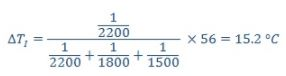Similarly, the temperature drop across ΙΙ-effect,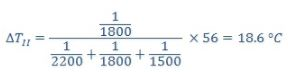And the temperature drop across ΙΙΙ-effect,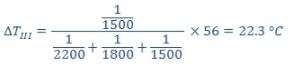Therefore, the boiling point in the first effect will be = (105 – 15.2) °C = 89.8 °C
Similarly, the boiling point in the second effect will be = (89.8 – 18.6)°C = 71.2 °C.

Offer running on EduRev: Apply code STAYHOME200 to get INR 200 off on our premium plan EduRev Infinity!

## Heat Transfer for Engg.

46 videos|60 docs|59 tests

,

,

,

,

,

,

,

,

,

,

,

,

,

,

,

,

,

,

,

,

,

;